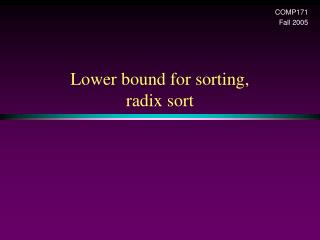Download PresentationLower bound for sorting, radix sort

# Lower bound for sorting, radix sort - PowerPoint PPT PresentationDownload Presentation## Lower bound for sorting, radix sort

- - - - - - - - - - - - - - - - - - - - - - - - - - - E N D - - - - - - - - - - - - - - - - - - - - - - - - - - -
##### Presentation Transcript

1. COMP171 Fall 2005 Lower bound for sorting,radix sort

2. Lower Bound for Sorting • Mergesort and heapsort • worst-case running time is O(N log N) • Are there better algorithms? • Goal: Prove that any sorting algorithm based on only comparisons takes (N log N) comparisons in the worst case (worse-case input) to sort N elements.

3. Lower Bound for Sorting • Suppose we want to sort N distinct elements • How many possible orderings do we have for N elements? • We can have N! possible orderings (e.g., the sorted output for a,b,c can be a b c, b a c, a c b, c a b, c b a, b c a.)

4. Lower Bound for Sorting • Any comparison-based sorting process can be represented as a binary decision tree. • Each node represents a set of possible orderings, consistent with all the comparisons that have been made • The tree edges are results of the comparisons

5. Decision tree for Algorithm X for sorting three elements a, b, c

6. Lower Bound for Sorting • A different algorithm would have a different decision tree • Decision tree for Insertion Sort on 3 elements:

7. Lower Bound for Sorting • The worst-case number of comparisons used by the sorting algorithm is equal to the depth of the deepest leaf • The average number of comparisons used is equal to the average depth of the leaves • A decision tree to sort N elements must have N! leaves • a binary tree of depth d has at most 2d leaves  the tree must have depth at least log2 (N!) • Therefore, any sorting algorithm based on only comparisons between elements requires at least  log2(N!)  comparisons in the worst case.

8. Lower Bound for Sorting • Any sorting algorithm based on comparisons between elements requires (N log N) comparisons.

9. Linear time sorting • Can we do better (linear time algorithm) if the input has special structure (e.g., uniformly distributed, every numbers can be represented by d digits)? Yes. • Counting sort, radix sort

10. Counting Sort • Assume N integers to be sorted, each is in the range 1 to M. • Define an array B[1..M], initialize all to 0  O(M) • Scan through the input list A[i], insert A[i] into B[A[i]]  O(N) • Scan B once, read out the nonzero integers  O(M) Total time: O(M + N) • if M is O(N), then total time is O(N) • Can be bad if range is very big, e.g. M=O(N2) N=7, M = 9, Want to sort 8 1 9 5 2 6 3 3 5 6 8 9 1 2 Output: 1 2 3 5 6 8 9

11. Counting sort • What if we have duplicates? • B is an array of pointers. • Each position in the array has 2 pointers: head and tail. Tail points to the end of a linked list, and head points to the beginning. • A[j] is inserted at the end of the list B[A[j]] • Again, Array B is sequentially traversed and each nonempty list is printed out. • Time: O(M + N)

12. Counting sort M = 9, Wish to sort 8 5 1 5 9 5 6 2 7 1 2 5 6 7 8 9 5 5 Output: 1 2 5 5 5 6 7 8 9

13. Radix Sort • Extra information: every integer can be represented by at most k digits • d1d2…dkwheredi are digits in base r • d1: most significant digit • dk: least significant digit

14. Radix Sort • Algorithm • sort by the least significant digit first (counting sort) => Numbers with the same digit go to same bin • reorder all the numbers: the numbers in bin 0 precede the numbers in bin 1, which precede the numbers in bin 2, and so on • sort by the next least significant digit • continue this process until the numbers have been sorted on all k digits

15. Radix Sort • Least-significant-digit-first Example: 275, 087, 426, 061, 509, 170, 677, 503

16. Radix Sort • Does it work? • Clearly, if the most significant digit of a and b are different and a < b, then finally a comes before b • If the most significant digit of a and b are the same, and the second most significant digit of b is less than that of a, then b comes before a.

17. Radix Sort Example 2: sorting cards • 2 digits for each card: d1d2 • d1 = : base 4 •        • d2 = A, 2, 3, ...J, Q, K: base 13 • A  2  3  ...  J  Q  K • 2  2  5  K

18. // base 10 // FIFO // d times of counting sort // scan A[i], put into correct slot // re-order back to original array

19. Radix Sort • Increasing the base r decreases the number of passes • Running time • k passes over the numbers (i.e. k counting sorts, with range being 0..r) • each pass takes O(N+r) • total: O(Nk+rk) • r and k are constants: O(N)